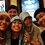I am still confused about limit?

If given $f(c) = L,$

(a) should $\lim_{x \to c} f(x)$ exist?

(b) If the limit is exist, should $\lim_{x \to c} f(x) = L?$

PS:

I don't know how can I prove it. But I know that $\lim_{x \to c} f(x) = L$ exist if and only if $\lim_{x \to c^+} f(x) = L$ and $\lim_{x \to c^-} f(x) = L.$ Hence (b) is not true. But then my teacher said that was an incomplete solution. Can anyone help?Note by Nabila Nida Rafida
6 years, 1 month ago

This discussion board is a place to discuss our Daily Challenges and the math and science related to those challenges. Explanations are more than just a solution — they should explain the steps and thinking strategies that you used to obtain the solution. Comments should further the discussion of math and science.

When posting on Brilliant:

• Use the emojis to react to an explanation, whether you're congratulating a job well done , or just really confused .
• Ask specific questions about the challenge or the steps in somebody's explanation. Well-posed questions can add a lot to the discussion, but posting "I don't understand!" doesn't help anyone.
• Try to contribute something new to the discussion, whether it is an extension, generalization or other idea related to the challenge.
• Stay on topic — we're all here to learn more about math and science, not to hear about your favorite get-rich-quick scheme or current world events.

MarkdownAppears as
*italics* or _italics_ italics
**bold** or __bold__ bold

- bulleted
- list

• bulleted
• list

1. numbered
2. list

1. numbered
2. list
Note: you must add a full line of space before and after lists for them to show up correctly
paragraph 1

paragraph 2

paragraph 1

paragraph 2

> This is a quote
This is a quote
# I indented these lines
# 4 spaces, and now they show
# up as a code block.

print "hello world"
# I indented these lines
# 4 spaces, and now they show
# up as a code block.

print "hello world"
MathAppears as
Remember to wrap math in $$...$$ or $...$ to ensure proper formatting.
2 \times 3 $2 \times 3$
2^{34} $2^{34}$
a_{i-1} $a_{i-1}$
\frac{2}{3} $\frac{2}{3}$
\sqrt{2} $\sqrt{2}$
\sum_{i=1}^3 $\sum_{i=1}^3$
\sin \theta $\sin \theta$
\boxed{123} $\boxed{123}$

Sort by:

Then limit must be continuous for limx→cf(x)=L.

- 6 years ago

To know the basics of limits, i suggest u to join a free online course of calculus one on coursera.org

- 6 years ago

(a) The function being defined at $c$ does not mean that the limit is defined at $c$. For example, given the piecewise function

$f(x) = \frac{1}{x} (x < 0)$ $f(x) = 5 - x(x = 0)$ $f(x) = sin(\frac{1}{x}) (x > 0)$

At 0, $f$ is defined (it equals 5), but the limit does not exist at 0 because the left and right sided limits are unequal (and don't exist).

(b) No. Again, with a piecewise function

$f(x) = \frac {x^2}{x} (x < 0)$ $f(x) = x + 3 (x = 0)$ $f(x) = x^2 (x > 0)$

The limit of this function at 0 is in fact 0 since the left sided limit is 0 as well as the right sided limit. However, when this function is defined at 0, it is not 0, in fact, it is 3.

Accompanying graph: https://i.imgur.com/2Gh4qJF.png

The enclosed red circle indicates $f(0)$, i.e., what the function is when evaluated at $0$. However, the empty red circle indicates the limit at 0, i.e., the mutual value that the functions to the left and right side of it are approaching.

- 6 years, 1 month ago

Wow, thanks for your quick answer Michael T. I answer the same, saying both questions with no. But I wonder, are there any proof without giving counterexample?

- 6 years, 1 month ago

Another example (if you want) of a function like above is: $f(x) = \left\{ \begin{array}{l l} 2 & \quad x = 0\\ 1 & \quad x \neq 0 \end{array} \right.$

Even though $\lim_{x \to 0} f(x) = 1$, f(0) is 2.

Hmmm I pose a question though. In the original question, the function didn't have to be continuous, but what if it did? If the function is continuous, would the statements above always be true? Would the $\lim_{x \to c} f(x) = L$ mean that $f(c) = L$ for continuous functions?

- 6 years, 1 month ago

I believe that's actually one of the definitions of a continuous function. I'm a bit rusty on that though.

- 6 years, 1 month ago

Yep, a function $f(x)$ is continuous iff $\lim_{x \to c} f(x) = f(c)$ for all $c$ (in the domain of $f.$)

- 6 years ago